# Civil Engineering - RCC Structures Design

### Exercise :: RCC Structures Design - Section 5

16.

If the length of a combined footing for two columns l metres apart is L and the projection on the left side of the exterior column is x, then the projection y on the right side of the exterior column, in order to have a uniformly distributed load, is (where x is the distance of centre of gravity of column loads).

 A. y = L - (l - x) B.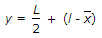C.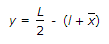D.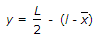Explanation:

No answer description available for this question. Let us discuss.

17.

The section of a reinforced beam where most distant concrete fibre in compression and tension in steel attains permissible stressess simultaneously, is called

 A. balanced section B. economic section C. critical section D. all the above.

Explanation:

No answer description available for this question. Let us discuss.

18.

The width of the flange of a L-beam, should be less than

 A. one-sixth of the effective span B. breadth of the rib + four times thickness of the slab C. breadth of the rib + half clear distance between ribs D. least of the above.

Explanation:

No answer description available for this question. Let us discuss.

19.

If the maximum dip of a parabolic tendon carrying tension P is h and the effective length of the prestressed beam is L, the upward uniform pressure will be

 A.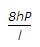B.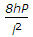C.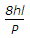D.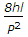E.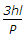Explanation:

No answer description available for this question. Let us discuss.

20.

A singly reinforced concrete beam of 25 cm width and 70 cm effective depth is provided with 18.75 cm2 steel. If the modular ratio (m) is 15, the depth of the neutral axis, is

 A. 20 cm B. 25 cm C. 30 cm D. 35 cm E. 40 cm